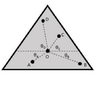# Determine the net torque acting about the center of mass

• paulimerci

#### paulimerci

Homework Statement
A triangle is suspended from the center of mass located at point O and has four 2 Kg mass suspended at
points A, B, C and D. The angles to points A, B, C and D are measured from the horizontal through the
center of mass where θA = 30 ̊ below, θB = 20 ̊ below, θC = 60 ̊ above and
θD = 80 ̊ above. The distances from point O to points A, B, C, and D are
20 cm, 35 cm, 15 cm and 30 cm respectively. Determine the net torque
acting about the center of mass.
Relevant Equations
T = F × r × sinθ
Net torque = 2gx20xcos30 - 2gx35xcos20 - 2gx15xcos60 + 2gx30xcos80
= -357Nm
Have I done it right?

#### Attachments

•Screen Shot 2022-10-30 at 6.07.18 AM.png
15.2 KB · Views: 35
It seems correct to me.
I got 3.50 N-m clockwise, by using g=9.81
I would have written 2g as a common factor.

Last edited:
•paulimerci
It seems correct to me.
I got 350.4 N-m clockwise, by using g=9.81
I would have written 2g as a common factor.
Thank you!

•Lnewqban
Remember the distances are given in cm, not metres.

(Edit- original message deleted.)

Remember the distances are given in cm, not metres.
Thanks, yes I should have done that. I used g = 10m/s2. And I did factoring out 2g in my worksheet but didn't include in the equation above.

Thanks, yes I should have done that. I used g = 10m/s2. And I did factoring out 2g in my worksheet but didn't include in the equation above.
Hi @paulimerci. If you haven't already spotted it, note that your answer (357Nm) is 100 times too big (because you haven't converted cm to metres).

Also, the data are supplied to 1 or 2 significant figures. Giving the final answer to more than 2 significant figures isn’t good practice.

•Lnewqban
Thanks for pointing out, hope I should not do this mistake again.

•Steve4Physics
Remember the distances are given in cm, not metres.

(Edit- original message deleted.)
Thank you, I have edited post #2.
Then, I got 3.50 N-m clockwise.

•paulimerci